# Conformal structure

A conformal structure on a vector spaceis a classof pairwise-homothetic Euclidean metrics on. Any Euclidean metricondefines a conformal structure,called the conformal structure induced by the Euclidean metric. An automorphismofis called an automorphism of the conformal structureif the induced transformation on the space of bilinear forms preserves the set. The group of automorphisms of a conformal structure is isomorphic to the linear conformal group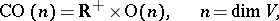which is the direct product of the multiplicative group of positive numbers and the orthogonal group.

A conformal structure on a manifold is a field of conformal structures on the tangent spaces, that is, a subbundle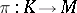of the bundle of symmetric bilinear forms on the manifoldwhose fibres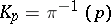are conformal structures on the corresponding tangent spaces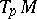. The bundleis topologically trivial and any sectionof it (giving rise to a Riemannian metric on) uniquely defines a conformal structure according to the formulaThe sectionis called a Riemannian metric subordinate to the conformal structure. Any other sectionof the bundle has the form, whereis a positive function on, that is, the Riemannian metrics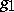andare conformally equivalent. Therefore a conformal structure can also be defined as a class of conformally-equivalent Riemannian metrics. A conformal structureon a manifoldcan be identified with the-structureonconsisting of all frames onthat are orthonormal with respect to at least one Riemannian metric that is subordinate to. The main properties of a conformal structure are determined by the fact that a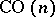-structureis a-structure of order two: Its first extension is an-structureon, while the second extension is an-structure (a field of frames) on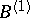. Hence, in particular, it follows that the group of automorphisms of(which is the same as the group of conformal transformations of any Riemannian metric subordinate to) is a Lie group of dimension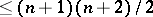, while the isotropy representation of its stationary subgroup in the tangent space of the second order is faithful.

As a rule, the group of automorphisms of a conformal structureis the same as the group of motions of some Riemannian metric subordinate to it. The only exceptions are the standard conformal structures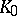on the sphere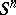and on the Euclidean space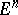, generated by the standard Riemannian metrics. A conformal structure on a manifoldis called locally flat if it is locally equivalent to the standard conformal structureof a Euclidean space, that is, if there exists in a neighbourhood of any pointa flat Riemannian metric subordinate to. In order that a conformal structure be locally flat it is necessary and sufficient that the Weyl conformal curvature tensor of some (and therefore of any) Riemannian metric subordinate to it be zero. Examples of locally flat conformal structures are the standard conformal structures in a Euclidean space, on the spheres, in a Lobachevskii space, and also in the spaces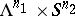and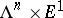generated by the standard metrics. All locally flat conformal structures on simply-connected manifolds with a transitive group of automorphisms are accounted for in this way. The standard conformal structureon the sphereis the only conformal structure having a maximal (in the sense of dimension) group of automorphisms. The sphereendowed with the conformal structureis called a conformal space.

The notion of a conformal structure is closely related to that of a conformal connection on: such a connection always defines a conformal structure on; on the other hand, a conformal connection is the connection in the reduced principal fibre bundle that is defined by the given conformal structure.